Calculus Problem Solver 1.0

 Product Overview How to use Screen shots Try it online

 Get it

### Solving Differentiation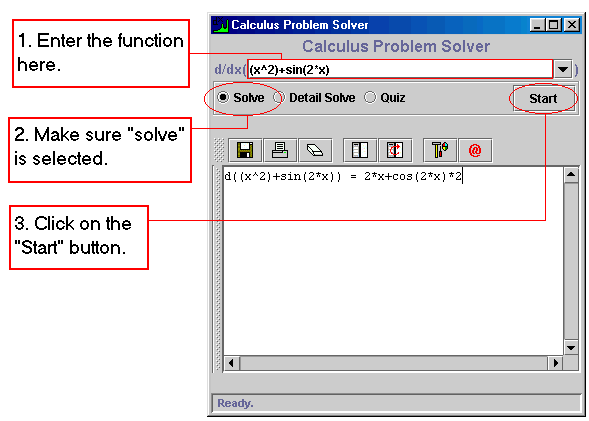### Valid Inputs

When entering the equations make sure the following general rules hold:

• The only valid variable is X (small or capital letter). Any other variable such as e, pi, var and etc will be considered as a constant.
• Multiplication sign (*) can not be omitted. (ie. 2x is invalid, 2*x is correct)
• Multiplication has higher priority than addition. Use parenthesis to avoid confusion. (ie. x+2*x is same as x+(2*x))

Examples Inputs:

• x+2*x
• (x+2)*x
• x^5
• 2^x
• e^x
• e^(x+5)+x^5
• sin(x+2*x)
• cos(x^2)
• tan(x*x)
• arcsin(2*x)
• arctan(x)

### Displaying Detailed Step-By-Step Solutions

The Detailed solutions generated by Calculus Problem Solver clearly demonstrate:

1. How the problem was divided into pieces;
2. What differentiation formulas have been used;
3. What values are substituted in the formulas;
4. How the partial solutions were substituted into each other to give final answer.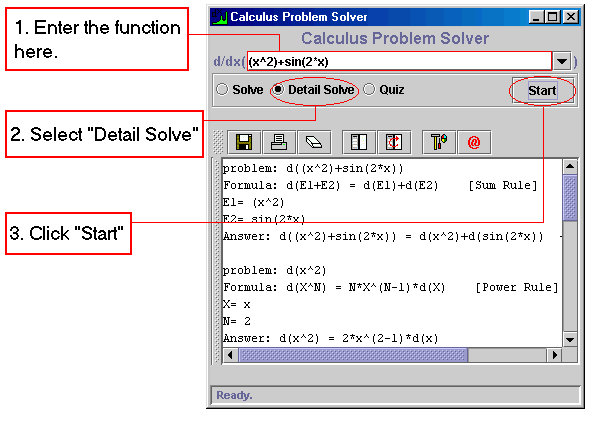### Practicing With Quiz

1. Type the input function;
2. Select "Quiz";
3. Press "Start".
4. Select the correct formula when prompted.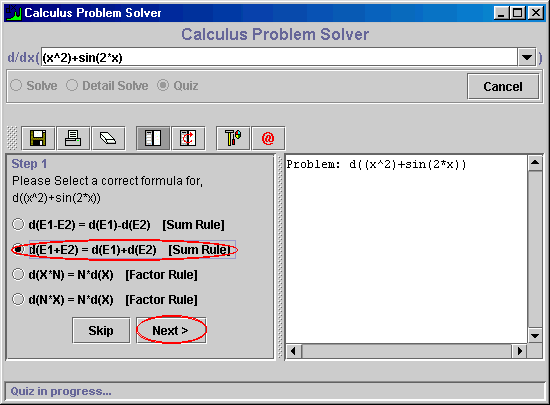If you select a wrong answer, you will be notified and will be given a chance to try again.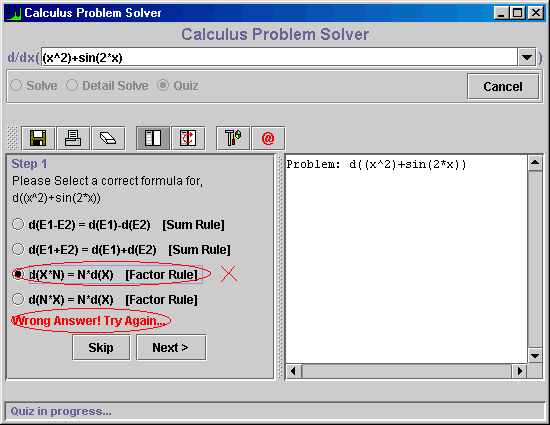Upon selecting a correct formula, you will be prompted to enter the correct substitutions in the formulas.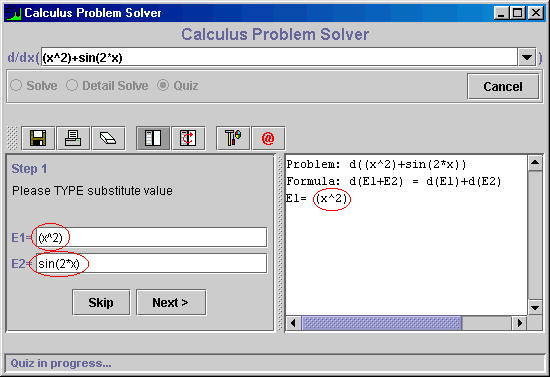Copyright (c) 2004 - Runiter Company - support@runiter.com